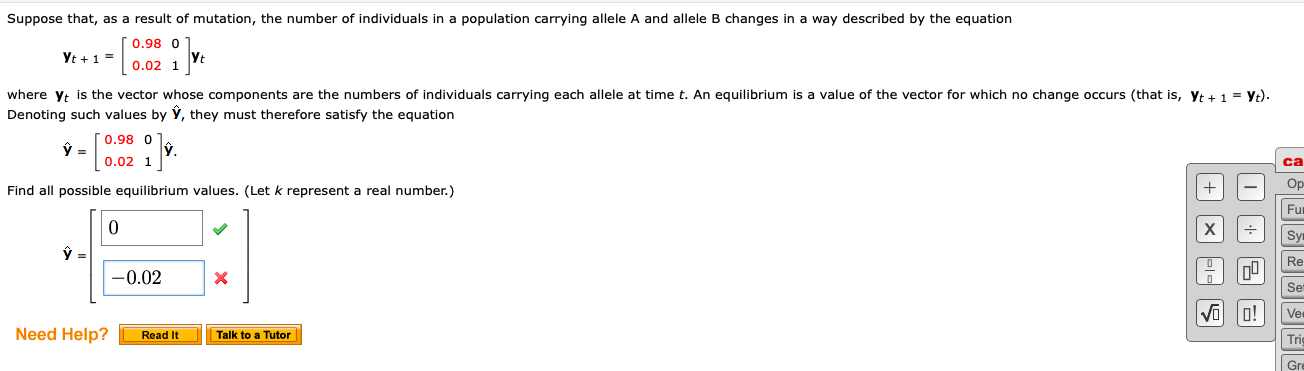# Question Solved1 Answerhelp please Suppose that, as a result of mutation, the number of individuals in a population carrying allele A and allele B changes in a way described by the equation [ 0.98 01 +1" 0.02 1 ] where Yt is the vector whose components are the numbers of individuals carrying each allele at time t. An equilibrium is a value of the vector for which no change occurs (that is, Yt + 1 = Yt). Denoting such values by Y, they must therefore satisfy the equation 0.98 0 0 0.02 1] Find all possible equilibrium values. (Let k represent a real number.) + + O 6 -0.02 S 9 Need Help? Read It Talk to a TutorTranscribed Image Text: Suppose that, as a result of mutation, the number of individuals in a population carrying allele A and allele B changes in a way described by the equation [ 0.98 01 +1" 0.02 1 ] where Yt is the vector whose components are the numbers of individuals carrying each allele at time t. An equilibrium is a value of the vector for which no change occurs (that is, Yt + 1 = Yt). Denoting such values by Y, they must therefore satisfy the equation 0.98 0 0 0.02 1] Find all possible equilibrium values. (Let k represent a real number.) + + O 6 -0.02 S 9 Need Help? Read It Talk to a Tutor
More
Transcribed Image Text: Suppose that, as a result of mutation, the number of individuals in a population carrying allele A and allele B changes in a way described by the equation [ 0.98 01 +1" 0.02 1 ] where Yt is the vector whose components are the numbers of individuals carrying each allele at time t. An equilibrium is a value of the vector for which no change occurs (that is, Yt + 1 = Yt). Denoting such values by Y, they must therefore satisfy the equation 0.98 0 0 0.02 1] Find all possible equilibrium values. (Let k represent a real number.) + + O 6 -0.02 S 9 Need Help? Read It Talk to a Tutor